Next: The Numerov algorithm for Up: Numerical treatment of the Previous: Numerical treatment of the

## The problem inside a box

The system of differential equations (34) is solved in coordinate representation in a spherical box for a given choice of boundary conditions. Here we briefly introduce notations that useful for the discussion that follows and discuss the validity of approximations to solve the differential problem. The radial coordinate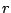is approximated by a mesh of points spaced by the step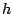: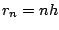, with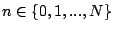. For any quantity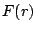approximated on the mesh we use notation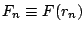.

On the wall of the box, we use two possible boundary conditions: vanishing wave functions (Dirichlet condition) or vanishing derivatives of the wave functions (Neumann condition). With this choice, the continuous part of the spectrum is discretized. Important questions are how well the continuum is approximated by this discretization and whether it does not introduce numerical artifacts when solving system of equations (34). A discussion concerning the behaviour of the resonant like solution inside the box can be found in . The non-linearity of the equations makes it difficult to discuss further the effect of the discretization of the spectrum, nevertheless, the numerical examples shown in Sec. 8 show that such numerical method provides a fairly good approximation of the exact problem. In particular, it can be observed that once a sufficiently large box is used, the global properties of the nucleus become perfectly independent of its size.Next: The Numerov algorithm for Up: Numerical treatment of the Previous: Numerical treatment of the
Jacek Dobaczewski 2005-01-23